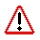CPSC 331 Operating Systems Fall 2005

# C/C++ Notes

This document is intended to provide a quick overview of some C and/or C++ language features which you will encounter during the Nachos projects and other parts of the course which may be unfamiliar to you.Additional material will be added as it becomes relevant.

## Passing Functions as Parameters

[C/C++]

C and C++ allow functions to be passed as parameters. This allows a degree of generic programming in which entire tasks (not just values!) are provided as parameters to a function. Nachos uses this feature in several places, including Thread::Fork and List::Apply.

#### Declaration

A prototype for a function which takes a function parameter looks like the following:

```void func ( void (*f)(int) );
```

This states that the parameter f will be a pointer to a function which has a void return type and which takes a single int parameter. The following function (print) is an example of a function which could be passed to func as a parameter because it is the proper type:

```void print ( int x ) {
cout << x << endl;
}
```

#### Function Call

When calling a function with a function parameter, the value passed must be a pointer to a function. Use the function's name (without parens) for this:

```func(print);
```

would call func, passing the print function to it.

#### Function Body

As with any parameter, func can now use the parameter's name in the function body to access the value of the parameter. Let's say that func will apply the function it is passed to the numbers 0-4. Consider, first, what the loop would look like to call print directly:

```for ( int ctr = 0 ; ctr < 5 ; ctr++ ) {
print(x);
}
```

Since func's parameter declaration says that f is the name for a pointer to the desired function, we recall first that if f is a pointer then *f is the thing that f points to (i.e. the function print in this case). As a result, just replace every occurrence of print in the loop above with *f:

```void func ( void (*f)(int) ) {
for ( int ctr = 0 ; ctr < 5 ; ctr++ ) {
(*f)(x);
}
}
```

The extra set of parens around *f is necessary because of the precedence of *: *f(x) would mean the thing that the value returned by f(x) points to.

#### Notes

There are some limitations - don't try to pass class methods as parameters. Use only free functions.

## void * Parameters

[C/C++]

One way to create functions which can work with a variety of parameter types is to declare the parameter to be of type void *, and then cast it to the appropriate type in the function body. In terms of type-checking, any pointer type matches void *.

This feature is used in Thread::Fork, for example, to allow threads to run any single-parameter function regardless of the type of the parameter. In this case, casting isn't necessary - the function which is passed to Fork can be declared to take a parameter of whatever type it requires (as long as it is a pointer type) which type-checks in comparison to the VoidFunctionPtr type.

## Inline Functions

[C++, in this context]

While typically the implementations of class methods are placed in the implementation (.cc) file, separate from the class definition in the header (.h) file, it is possible to provide method bodies directly in the class definition. These are known as inline functions. For example:

```class Dice {

public:
Dice ();
int roll ();
int numSides () const { return numSides_; }

private:
int numSides_;

};
```

numSides is an inline function because the body is given directly in the class definition.

Inline functions are used for efficiency. The compiler pastes a copy of the inlined function body in place of the function call, speeding up execution (by saving the overhead of making a function call) at the cost of increased program size (because the function body is copied in many places). It is generally poor form to inline functions that are longer than a few lines because it makes the source code harder to read and can significantly increase the size of the executable.

## Call-by-Reference or Pointer?

[C/C++]

If you want to pass a potentially large something to a function as a parameter and want to allow the function to modify the something if it wishes, what do you do? You could use call-by-reference e.g.:

```void func ( SomeType & param );
```

Or, you could pass a pointer to the thing:

```void func ( SomeType * param );
```

Neither method copies the object, saving time and allow the function to modify it. So which to use? Nachos uses pointers instead of call-by-reference in many circumstances. In some cases, this is so void * parameter types can be used to achieve generic programming.

 last updated: --Mon Oct 10 15:37:22 EDT 2005-- page owned by: bridgeman@hws.edu